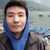## TyroCity is a community of amazing learners

We're a place where students share, stay up-to-date, learn and grow.

# Discussion on: About Marginal cost of capitalMarginal Cost

"Though there may be differences as to its exact method of calculation, there is general agreement that the marginal cost of capital is an important criterion when making investment decisions. An integral part of this computation is the company’s retained earnings. If the objective of the marginal cost of capital is to serve as a criterion for the investment decision, the computed marginal cost of capital should reflect its intended value. (Hollinger, 1978)” Marginal cost of capital is the cost incurred in producing one additional unit of product or service. This is calculated as

Marginal cost = change in costs/change in quantity

The change is cost is calculated by deducting the total cost with cost incurred before production of additional unit. Similarly, the change in quantity is calculated as total quantity minus the previous quantity before additional quantity. This cost calculates the cost related with one additional unit and is used to evaluate how much production will make the companies cost of producing the lowest considering the economies of scale.

Marginal cost usually calculated the operating cost of production of a unit as the fixed asset is already there and will not incur cost. Therefore to get the actual marginal cost, calculation are done for a longer period so fixed assets are also a part of marginal cost.

It is calculated as

WACC = (wd)(kd)(1-t) + (wps)(kps) + (wce)(kce)

The WACC calculates the average cost of capital by taking the weight of all the variables involved.

Historic cost of capital

It is also known as the net cash outlay (NCO). When a company invests in a project or service, it invests through either debt or equity. The return from investment is expected to be higher than the NCO. Thus, the required rate of return forms a benchmark to be met by the investment. This takes into consideration of the risk factor. Higher the risk higher the expected rate of return. ‘Cost of Capital’ is relevant while structuring the capital requirements of a company, for deciding the right mix of means of financing and for assessing the success or otherwise of any project. It forms the basis of return any investment has to earn so as to maximize shareholder wealth. (Gopalan, 2011)

This cost of capital is a projection for future. Therefore the data taken from past history is only relevant for project to be done from inside the company. So for projects outside the company, the cost of capital generated from history of the company will not work.

A company’s investment generally includes both debt and equity so it is necessary to calculate both the cost of debt and cost of equity to find the cost of capital. The cost of debt from past history may not be relevant for future forecasting as the rate and type of debt may vary in the future.

It is calculated as

Cost of capital = Cost of debt + Cost of equity

Cost of debt (kd) = C/Po

Where, C = Coupon Amount and Po = Current Market price

Cost of Equity (Re) = Rm + Rf * B

Where Rm = Market risk premium, Rf = Risk free rate and B = beta risk

Thus marginal cost calculates the cost of additional cost of production which is a better method to calculate investing in securities rather than cost of capital that takes historic data which may not be relevant in future.

References

Gopalan, V. (2011, March). Relevance of ‘Cost of Capital’ in investment decision making. Management Accountant; Calcutta , 217-218.

Hollinger, R. D. (1978, April). The marginal cost of capital and retained earnings. Financial Executive; New York , p. 56.